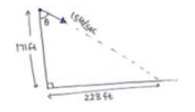# A person standing on top of a cliff

Question:

A person standing on top of a cliff $171 \mathrm{ft}$ high has to throw a packet to his friend standing on the ground $228 \mathrm{ft}$ horizontally away. If he throws the packet directly aiming at the friend with a speed of $15.0 \mathrm{ft} / \mathrm{s}$, how short will the packet fall?

Solution:

$\operatorname{Tan}_{\theta=53^{\circ}} \theta=228 / 171=4 / 3$$x$-axis $y$-axis

$u_{x}=15 \sin 53 u_{y}=15 \cos 53$

$u_{x}=12 \mathrm{ft} /$ sec $u_{y}=9 \mathrm{ft} / \mathrm{sec}$

$\mathrm{ay}_{\mathrm{y}}=32 \mathrm{ft} / \mathrm{sec}^{2}$

$s_{y}=171 \mathrm{ft}$

$s_{y}=u_{y} t+\frac{1}{2} a_{y} t^{2}$

$t=3 s e c$

$s_{x}=12 \times 3$

$=36 \mathrm{ft}$

Packet will fall short by $=228-36=192 \mathrm{ft}$